Related Articles

# Section formula – Internal and External Division | Coordinate Geometry

• Difficulty Level : Hard
• Last Updated : 27 Oct, 2020

Suppose a point divides a line segment into two parts which may be equal or not, with the help of the section formula we can find that point if coordinates of a line segment are given and we can also find the ratio in which the point divides the given line segment if coordinates of that point are given.

When a point C divides a line segment AB in the ratio m:n, then we use the section formula to find the coordinates of that point. The section formula has 2 types. These types depend on point C which can be present between the points or outside the line segment.

The two types are:

1. Internal Section Formula
2. External Section Formula

## Internal Section Formula

When the point divides the line segment in the ratio m : n internally at point C then that point lies in between the coordinates of the line segment then we can use this formula. It is also called Internal Division.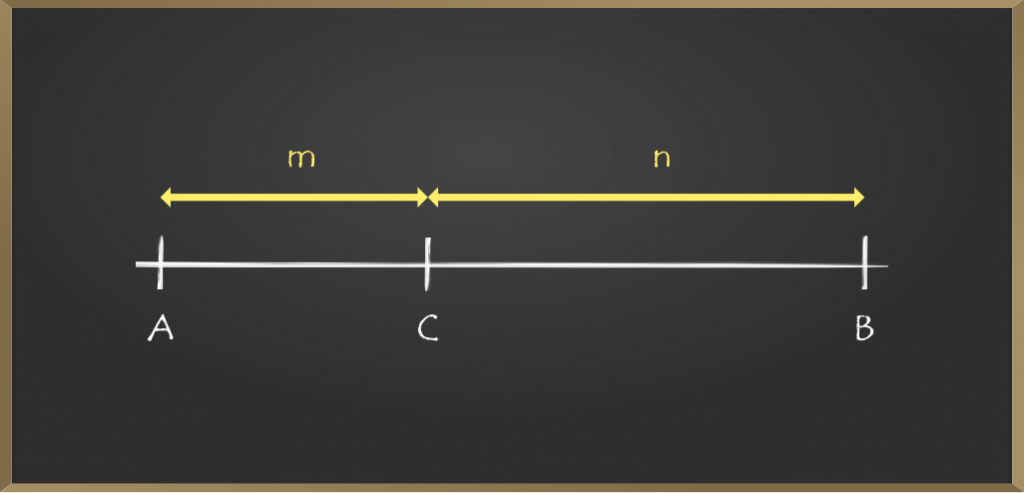If the coordinates of A and B are (x1, y1) and (x2, y2) respectively then Internal Section Formula is given as: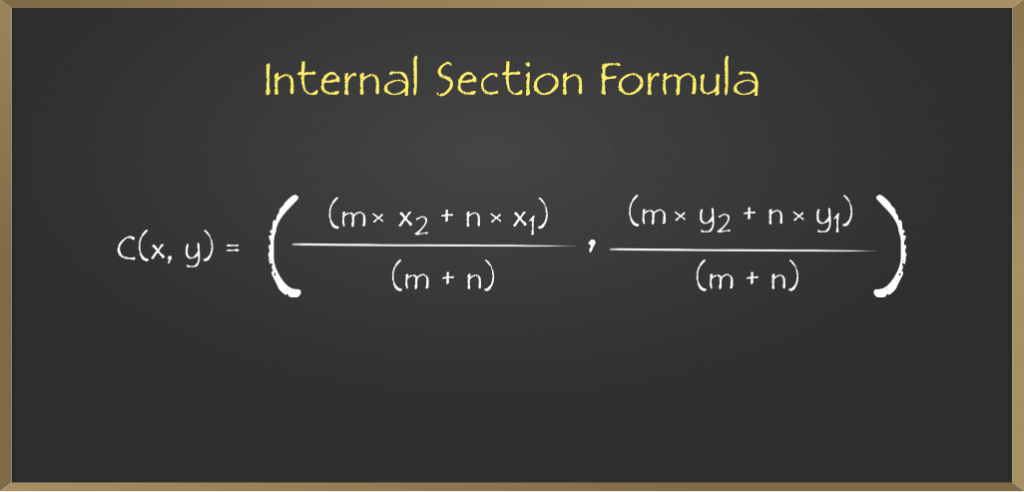### Derivation of the Formula

Let A (x1, y1) and B (x2, y2) be the endpoints of the given line segment AB and C(x, y) be the point which divides AB in the ratio m : n.

Then, AC / CB = m / n

We want to find the coordinates (x, y) of C.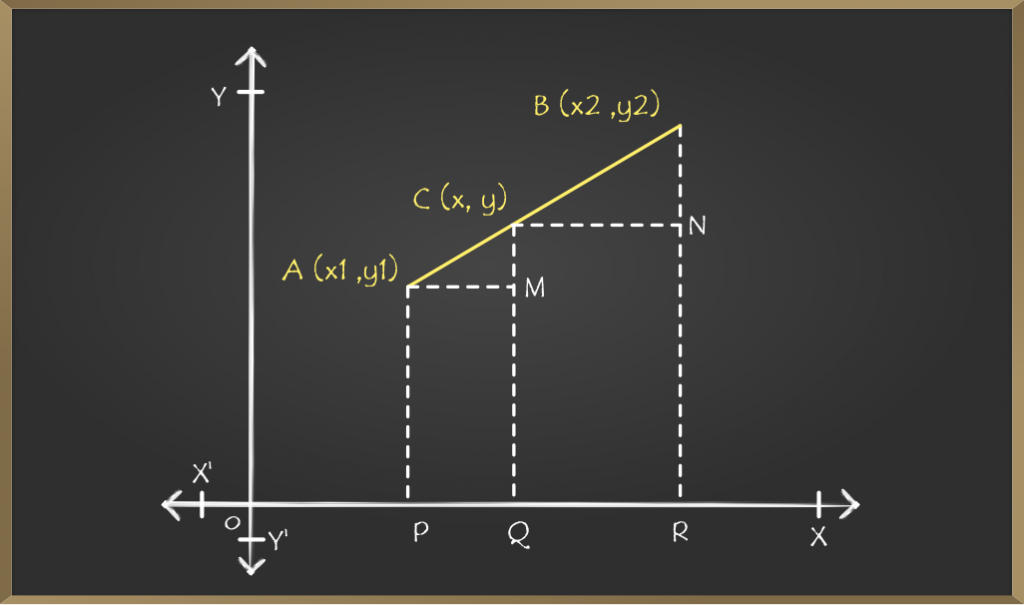Now draw perpendiculars of A, C, B parallel to Y coordinate joining at P, Q, and R on X-axis.

By seeing the above diagram,

AM = PQ = OQ – OP = (x – x1)

CN = QR = OR – OQ = (x2 –  x)

CM = CQ – MQ = (y – y1)

BN = BR – NR = (y2 – y)

Clearly, we can see that AMC and CNB are similar and, therefore, their sides are proportional by AA congruence rule.

AC / CB = AM / CN = CM / BN

Now substituting the values in the above relation

=> m / n = [x – x1 / x2 -x] = [y – y1 / y2 – y]

=> m / n = [x – x1 / x2 -x]  and m / n = [y – y1 / y2 – y]

Solving the 1st condition,

=> m(x2 – x) = n(x – x1)

=> (m + n)x = (mx2 + nx1)

=> x = (mx2 + nx1) / (m + n)

Solving the 1st condition,

=> m(y2 – y) = n(y – y1)

=> (m + n)y = (my2 + ny1)

=> y = (my2 + ny1) / (m + n)

Therefore, the co-ordinates of C (x, y) are

{ (m × x2 + n × x1) / (m + n ) ,  (m × y2 + n × y1) / (m + n ) }

## External Section Formula

When the point which divides the line segment is divided externally in the ratio m : n lies outside the line segment i.e when we extend the line it coincides with the point, then we can use this formula. It is also called External Division.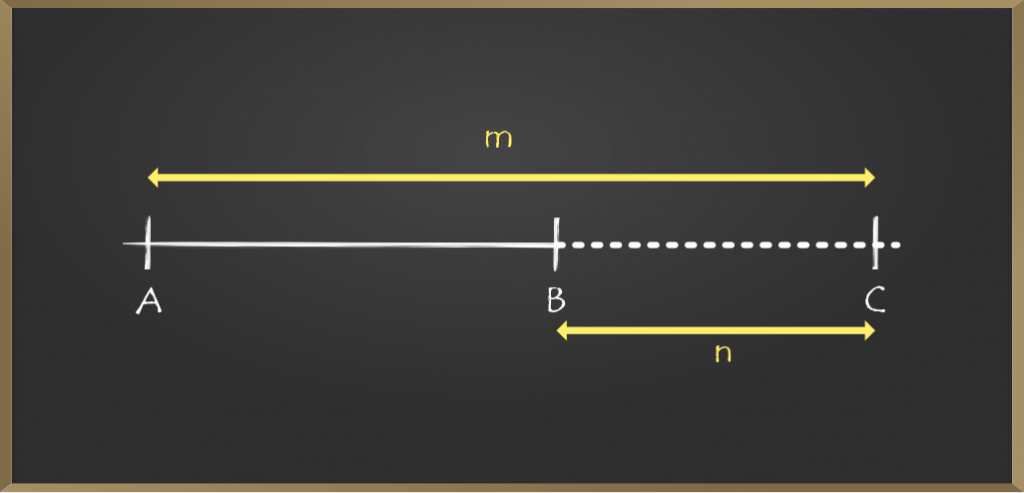If the coordinates of A and B are (x1,y1) and (x2,y2) respectively then external Section Formula is given as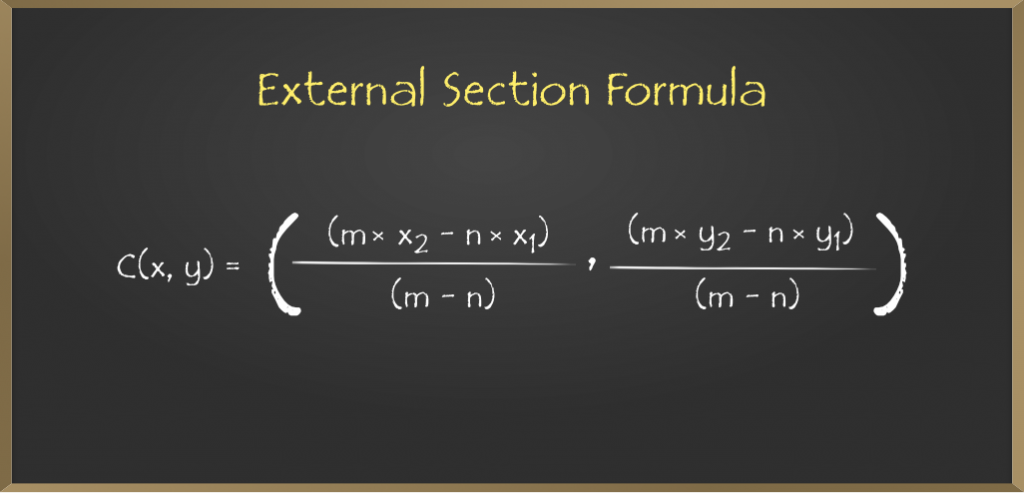### Derivation of the Formula

To derive the internal section we took a line segment and a point C(x, y) inside the line, but in the case of the external section formula, we have to take that point C(x, y) outside the line segment.

Let A(x1, y1) and B(x2, y2) be the endpoints of the given line segment AB and C(x, y) be the point which divides AB in the ratio m : n externally.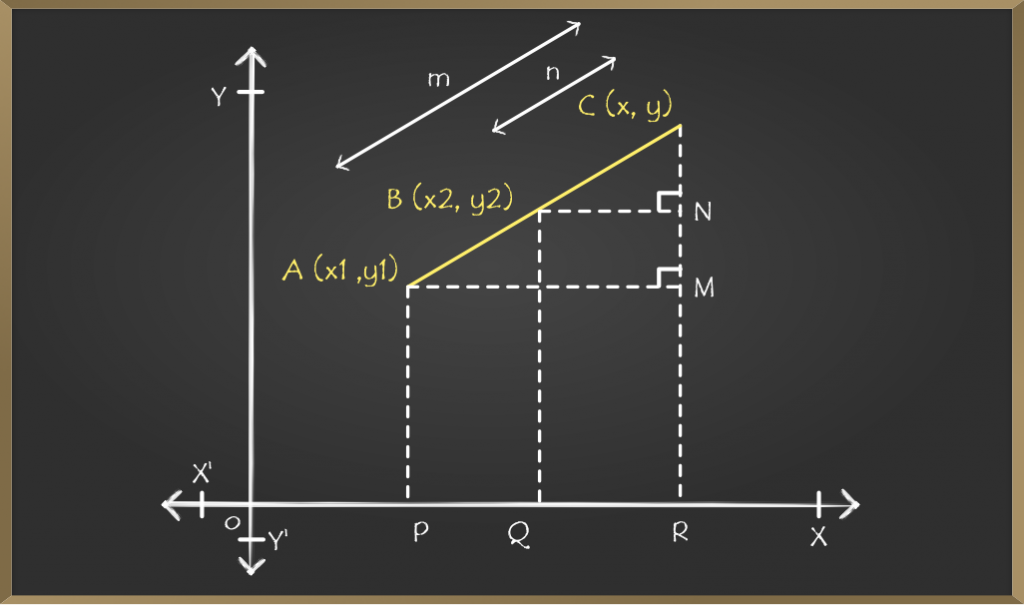We want to find the coordinates (x, y) of C. For that, draw perpendiculars of A, B, C parallel to Y coordinate joining at P, Q, and R on X axis.

By seeing the above diagram,

AM = PR = OR – OP = (x – x1)

BN = QR = OR – OQ = (x – x2)

similarly,

CM = RC – MR = (y – y1)

CN = CR – NR = (y – y2)

Clearly, we can see that triangle AMC and triangle BNC are similar and, therefore, their sides are proportional by AA congruence rule

AC / BC = AM / BN = CM / CN

Now substituting the values in the above relation

=> m / n = [x – x1 / x – x2] = [y – y1 / y – y2]

=> m / n = [x – x1 / x – x2] and m / n = [y – y1 / y – y2]

Solving the 1st condition,

=> m(x – x2) = n(x – x1)

=> (m – n)x = (mx2 – nx1)

=> x = (mx2 – nx1) / (m – n)

Solving the 2nd condition,

=> m(y – y2) = n(y – y1)

=> (m – n)y = (my2 – ny1)

=> y = (my2 – ny1) / (m – n)

Therefore, the co-ordinates of C (x, y) are

{ (m × x2 – n × x1) / (m – n) ,  (m × y2 – n × y1) / (m – n ) }

## Problems On Section Formula

Problem 1: Find the coordinates of point C (x, y) where it divides the line segment joining (4, – 1) and (4, 3) in the ratio 3 : 1 internally ?

Solution: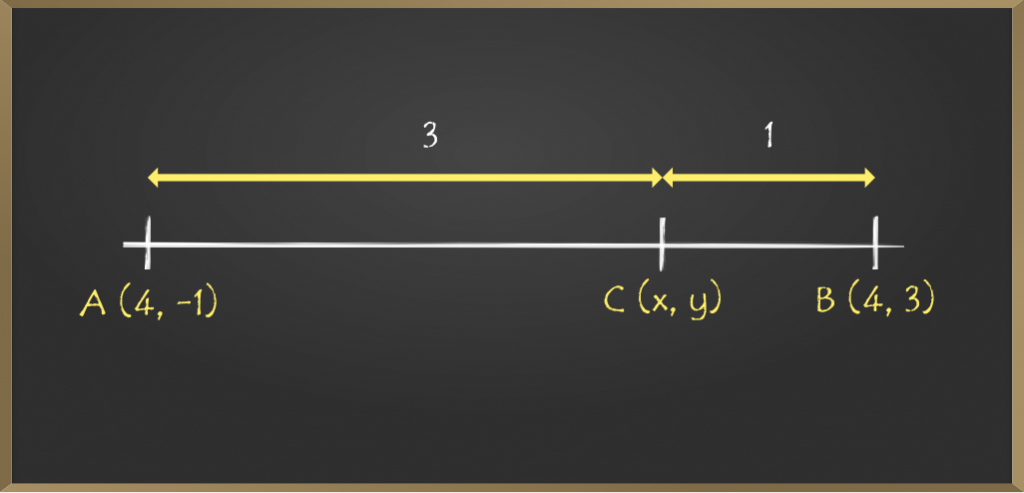Given coordinates are A (4, -3) and B (8, 5)

Let C (x, y) be a point which divides the line segment in the ratio of 3 : 1  i.e m : n = 3 : 1

Now using the formula C(x, y) = { (m × x2 + n × x1) / (m + n ) ,  (m × y2 + n × y1) / (m + n ) } as C is dividing internally.

=> C(x, y) = {(3*4 + 1*4 ) / (3+1), (3 * 3  + 1 *(-1)) / (3+1)}

=> C(x, y) = {16 / 4, 8 / 4}

=> C(x, y) = {4, 2}

Hence, the coordinates are (4, 2).

Problem 2: If a point P(k, 7) divides the line segment joining A(8, 9) and B(1, 2) in a ratio m : n then find values of m and n.

Solution:

It is not mentioned that the point is dividing the line segment internally or externally. So, at that time we will consider the internal section as the default.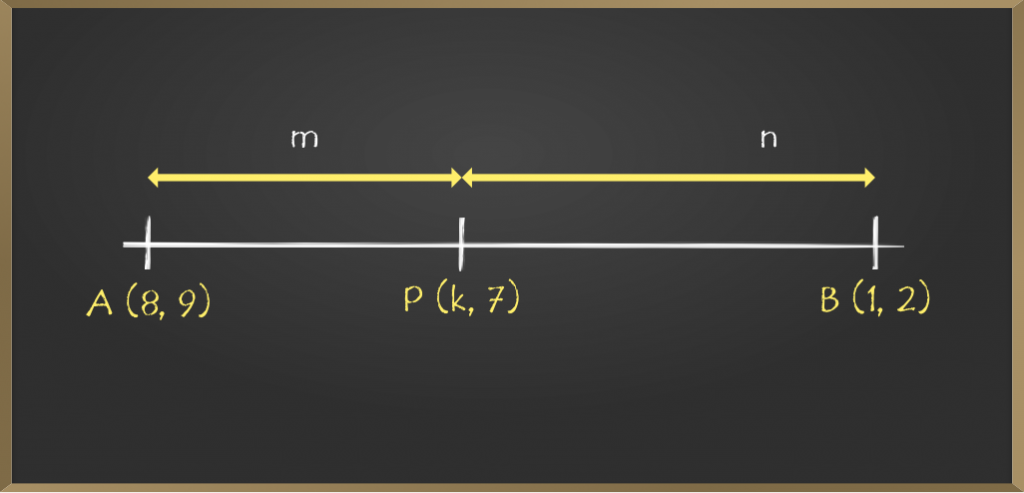Given coordinates are A (8, 9) and B (1, 2)

Let the given point P (k, 7) divides the line segment in the ratio of m : 1

Now using the section formula, finding only the x coordinate,

=> k = (m × x2 + n × x1) / (m + n )

=> k = (m × 1 + 1 × 8) / (m +1)

=> k = (m + 8) / (m + 1)

=> km + k = m + 8       …….(1)

Again using section formula for y coordinate.

=> 7 =  (m × y2 + n × y1) / (m + n )

=> 7 = (m × 2 + 1 × 9) / (m + 1)

=> 7 = (2m + 9) / (m +1)

=> 7m + 7 = 2m +9

=> 5m = 2

=> m = 5 / 2

So the required ratio is 5 : 2

Therefore, value of m is 5 and value of n is 2

Problem 3: A (4, 5) and B (7, -1) are two given points, and the point C divides the line-segment AB externally in the ratio 4 : 3. Find the coordinates of C.

Solution: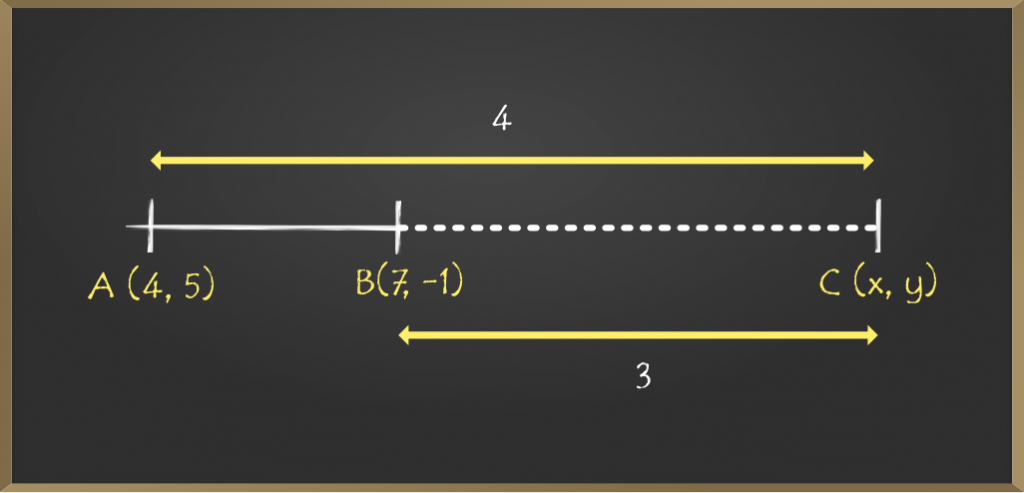Given coordinates are A (4, 5) and B (7, -1)

Let C (x, y) be a point which divides the line segment externally in the ratio of 4 : 3  i.e m : n = 4 : 3

Now using the formula C(x, y) = { (m × x2 – n × x1) / (m – n) ,  (m × y2 – n × y1) / (m – n ) } as C is dividing internally.

value of x = (mx2 – nx1) / (m – n)

=> (4 * 7 – 3 * 4) / (4 – 3)

=> 16

value of y = (my2 – ny1) / (m – n )

=> (4 * (-1) – 3 * 5) / (4 – 3)

=> -19

Hence, the coordinates are (16, -19).

Problem 4: Line 2x+y−4=0 divides the line segment joining the points A(2,−2) and B(3,7). Find the ratio of line segment in which the line is dividing?

Solution: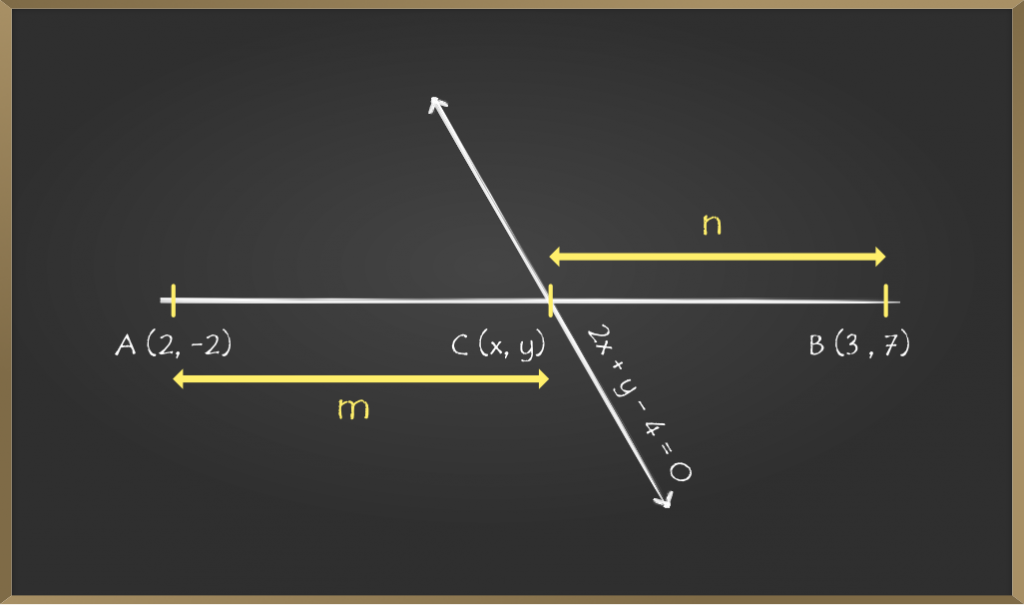Given coordinates are A (2, -2) and B (3, 7).

Line with equation 2x + y – 4 = 0 divides the line segment at point C (x, y)

Let us assume the given line cuts the line segment in the ratio 1 : n.

By section formula,

=> x = (m * x2 + n * x1) / (m + n)

=> x = (3 + 2n) / (1 + n)       ………..1

Similarly,

=> y = (m * y2 + n * y1) / (m + n)

=> y = (7 – 2n) / (1 + n)         ……….2

Now substituting the equations 1 and 2 in the given equation of the line.

=> 2x + y – 4 = 0

=> 2 [(3 + 2n) / (1 + n) ] +  [(7 – 2n) / (1 + n)] – 4 = 0

=> 6 + 4n + 7 − 2n − 4(1 + n) = 0

=>13 + 2n − 4 − 4n = 0

=>9 − 2n = 0

=> n = 2 / 9

Therefore the ratio at which the line divides is 9 : 2. We can also find the values of x and y by substituting the value of n in the equation 1 and 2.

Problem 5: A(2, 7) and B(–4, –8) are coordinates of the line segment AB. There are two points that trisected the segment. Find the coordinates of them.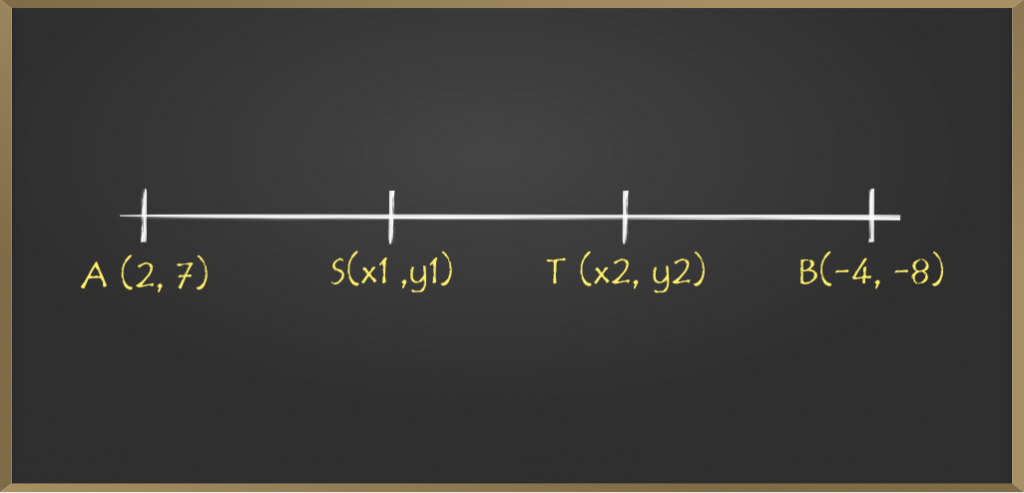Solution:

The two points trisected the line segment, which means the segment is divided into 3 equal parts.

AS = ST = TB                …………1

=> AS / SB

=> AS / ST + TB

=> AS / (AS + AS)          from equation 1

=> AS / 2 AS

=> 1 / 2

So, S divides the line segment AB in the ratio of 1 : 2

Now applying section formula to find the coordinates of point S

=> x1 = (1 × (-4) + 2 × 2) / (1 + 2)

=> x1 = (-4 + 4) / 3

=> x1 = 0

Similarly, for y coordinate,

=> y1 =  (1 × (-8) + 2 × 7) / (1 + 2)

=> y1 = (14 – 8) / 3

=> y1 = 2

Also.

=> AT / TB

=> (AS +ST) / TB

=> 2 TB / TB           from equation 1

=> 2 / 1

So, T divides the line segment AB in the ratio of 2 : 1

Now applying section formula to find the coordinates of point T

=> x2 = (2 × (-4) + 1 × 2) / (2 + 1)

=> x2 = (-8 + 2) / 3

=> x2 = -2

Similarly, for y coordinate

=> y2 =  (2 × (-8) + 1 × 7) / (2 + 1)

=> y2 = (-16 + 7) / 3

=> y2 = -3

Thus, the coordinates are :

S (x1, y1) = (0, 2)

T (x2, y2) = (-2, -3)

Attention reader! Don’t stop learning now. Join the First-Step-to-DSA Course for Class 9 to 12 students , specifically designed to introduce data structures and algorithms to the class 9 to 12 students

My Personal Notes arrow_drop_up